xOrdinal numberEncyclopediaIn set theory
Set theory
Set theory is the branch of mathematics that studies sets, which are collections of objects. Although any type of object can be collected into a set, set theory is applied most often to objects that are relevant to mathematics...

, an ordinal number, or just ordinal, is the order type
Order type
In mathematics, especially in set theory, two ordered sets X,Y are said to have the same order type just when they are order isomorphic, that is, when there exists a bijection f: X → Y such that both f and its inverse are monotone...

of a well-ordered set
Well-order
In mathematics, a well-order relation on a set S is a strict total order on S with the property that every non-empty subset of S has a least element in this ordering. Equivalently, a well-ordering is a well-founded strict total order...

. They are usually identified with hereditarily
Hereditary property
In mathematics, a hereditary property is a property of an object, that inherits to all its subobjects, where the term subobject depends on the context. These properties are particularly considered in topology and graph theory.-In topology:...

transitive set
Transitive set
In set theory, a set A is transitive, if* whenever x ∈ A, and y ∈ x, then y ∈ A, or, equivalently,* whenever x ∈ A, and x is not an urelement, then x is a subset of A....

s. Ordinals are an extension of the natural number
Natural number
In mathematics, the natural numbers are the ordinary whole numbers used for counting and ordering . These purposes are related to the linguistic notions of cardinal and ordinal numbers, respectively...

s different from integer
Integer
The integers are formed by the natural numbers together with the negatives of the non-zero natural numbers .They are known as Positive and Negative Integers respectively...

s and from cardinal
Cardinal number
In mathematics, cardinal numbers, or cardinals for short, are a generalization of the natural numbers used to measure the cardinality of sets. The cardinality of a finite set is a natural number – the number of elements in the set. The transfinite cardinal numbers describe the sizes of infinite...

s. Like other kinds of numbers, ordinals can be added, multiplied, and exponentiated.

Ordinals were introduced by Georg Cantor
Georg Cantor
Georg Ferdinand Ludwig Philipp Cantor was a German mathematician, best known as the inventor of set theory, which has become a fundamental theory in mathematics. Cantor established the importance of one-to-one correspondence between the members of two sets, defined infinite and well-ordered sets,...

in 1897 to accommodate infinite sequences and to classify sets with certain kinds of order
Order theory
Order theory is a branch of mathematics which investigates our intuitive notion of order using binary relations. It provides a formal framework for describing statements such as "this is less than that" or "this precedes that". This article introduces the field and gives some basic definitions...

structures on them. He discovered them by accident while working on a problem concerning trigonometric series--see Georg Cantor
Georg Cantor
Georg Ferdinand Ludwig Philipp Cantor was a German mathematician, best known as the inventor of set theory, which has become a fundamental theory in mathematics. Cantor established the importance of one-to-one correspondence between the members of two sets, defined infinite and well-ordered sets,...

.

The finite ordinals (and the finite cardinals) are the natural numbers: 0, 1, 2, …, since any two total orderings of a finite set are order isomorphic. The least infinite ordinal is ω, which is identified with the cardinal number ℵ0. However in the transfinite case, beyond ω, ordinals draw a finer distinction than cardinals on account of their order information. Whereas there is only one countably infinite cardinal, namely ℵ0 itself, there are uncountably many countably infinite ordinals, namely
ω, ω + 1, ω + 2, …, ω·2, ω·2 + 1, …, ω2, …, ω3, …, ωω, …, ωωω, …, ε0, ….

Here addition and multiplication are not commutative: in particular 1 + ω is ω rather than ω + 1 and likewise, 2·ω is ω rather than ω·2. The set of all countable ordinals constitutes the first uncountable ordinal ω1
First uncountable ordinal
In mathematics, the first uncountable ordinal, traditionally denoted by ω1 or sometimes by Ω, is the smallest ordinal number that, considered as a set, is uncountable. It is the supremum of all countable ordinals...

, which is identified with the cardinal ℵ1 (next cardinal after ℵ0). Well-ordered cardinals are identified with their initial ordinals, i.e. the smallest ordinal of that cardinality. The cardinality of an ordinal defines a many to one association from ordinals to cardinals.

In general, each ordinal α, is the order type of the set of ordinals strictly less than the ordinal, α itself. This property permits every ordinal to be represented as the set of all ordinals less than it. Ordinals may be categorized as: zero, successor ordinals, and limit ordinals (of various cofinalities
Cofinality
In mathematics, especially in order theory, the cofinality cf of a partially ordered set A is the least of the cardinalities of the cofinal subsets of A....

). Given a class of ordinals, one can identify the α-th member of that class, i.e. one can index (count) them. Such a class is closed and unbounded if its indexing function is continuous and never stops. The Cantor normal form uniquely represents each ordinal as a finite sum of ordinal powers of ω. However, this cannot form the basis of a universal ordinal notation due to such self-referential representations as ε0 = ωε0. Larger and larger ordinals can be defined, but they become more and more difficult to describe. Any ordinal number can be made into a topological space
Topological space
Topological spaces are mathematical structures that allow the formal definition of concepts such as convergence, connectedness, and continuity. They appear in virtually every branch of modern mathematics and are a central unifying notion...

by endowing it with the order topology
Order topology
In mathematics, an order topology is a certain topology that can be defined on any totally ordered set. It is a natural generalization of the topology of the real numbers to arbitrary totally ordered sets...

; this topology is discrete if and only if the ordinal is a countable cardinal, i.e. at most ω. A subset of ω + 1 is open in the order topology if and only if either it is cofinite
Cofinite
In mathematics, a cofinite subset of a set X is a subset A whose complement in X is a finite set. In other words, A contains all but finitely many elements of X...

or it does not contain ω as an element.

## Ordinals extend the natural numbers

A natural number
Natural number
In mathematics, the natural numbers are the ordinary whole numbers used for counting and ordering . These purposes are related to the linguistic notions of cardinal and ordinal numbers, respectively...

(which, in this context, includes the number 0
0 (number)
0 is both a numberand the numerical digit used to represent that number in numerals.It fulfills a central role in mathematics as the additive identity of the integers, real numbers, and many other algebraic structures. As a digit, 0 is used as a placeholder in place value systems...

) can be used for two purposes: to describe the size of a set, or to describe the position of an element in a sequence. When restricted to finite sets these two concepts coincide; there is only one way to put a finite set into a linear sequence, up to isomorphism. When dealing with infinite sets one has to distinguish between the notion of size, which leads to cardinal number
Cardinal number
In mathematics, cardinal numbers, or cardinals for short, are a generalization of the natural numbers used to measure the cardinality of sets. The cardinality of a finite set is a natural number – the number of elements in the set. The transfinite cardinal numbers describe the sizes of infinite...

s, and the notion of position, which is generalized by the ordinal numbers described here. This is because, while any set has only one size (its cardinality), there are many nonisomorphic well-orderings of any infinite set, as explained below.

Whereas the notion of cardinal number is associated with a set with no particular structure on it, the ordinals are intimately linked with the special kind of sets that are called well-order
Well-order
In mathematics, a well-order relation on a set S is a strict total order on S with the property that every non-empty subset of S has a least element in this ordering. Equivalently, a well-ordering is a well-founded strict total order...

ed (so intimately linked, in fact, that some mathematicians make no distinction between the two concepts). A well-ordered set is a totally ordered set (given any two elements one defines a smaller and a larger one in a coherent way) in which there is no infinite decreasing sequence (however, there may be infinite increasing sequences); equivalently, every non-empty subset of the set has a least element. Ordinals may be used to label the elements of any given well-ordered set (the smallest element being labeled 0, the one after that 1, the next one 2, "and so on") and to measure the "length" of the whole set by the least ordinal that is not a label for an element of the set. This "length" is called the order type of the set.

Any ordinal is defined by the set of ordinals that precede it: in fact, the most common definition of ordinals identifies each ordinal as the set of ordinals that precede it. For example, the ordinal 42 is the order type of the ordinals less than it, i.e., the ordinals from 0 (the smallest of all ordinals) to 41 (the immediate predecessor of 42), and it is generally identified as the set {0,1,2,…,41}. Conversely, any set (S) of ordinals that is downward-closed—meaning that for any ordinal α in S and any ordinal β < α, β is also in the set—is (or can be identified with) an ordinal.

So far we have mentioned only finite ordinals, which are the natural numbers. But there are infinite ones as well: the smallest infinite ordinal is ω, which is the order type of the natural numbers (finite ordinals) and that can even be identified with the set of natural numbers (indeed, the set of natural numbers is well-ordered—as is any set of ordinals—and since it is downward closed it can be identified with the ordinal associated with it, which is exactly how we define ω).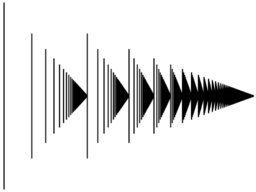Perhaps a clearer intuition of ordinals can be formed by examining a first few of them: as mentioned above, they start with the natural numbers, 0, 1, 2, 3, 4, 5, … After all natural numbers comes the first infinite ordinal, ω, and after that come ω+1, ω+2, ω+3, and so on. (Exactly what addition means will be defined later on: just consider them as names.) After all of these come ω·2 (which is ω+ω), ω·2+1, ω·2+2, and so on, then ω·3, and then later on ω·4. Now the set of ordinals we form in this way (the ω·m+n, where m and n are natural numbers) must itself have an ordinal associated with it: and that is ω2. Further on, there will be ω3, then ω4, and so on, and ωω, then ωω², and much later on ε0 (epsilon nought
Epsilon nought
In mathematics, the epsilon numbers are a collection of transfinite numbers whose defining property is that they are fixed points of an exponential map. Consequently, they are not reachable from 0 via a finite series of applications of the chosen exponential map and of "weaker" operations like...

) (to give a few examples of relatively small—countable—ordinals). We can go on in this way indefinitely far ("indefinitely far" is exactly what ordinals are good at: basically every time one says "and so on" when enumerating ordinals, it defines a larger ordinal). The smallest uncountable ordinal is the set of all countable ordinals, expressed as ω1.

### Well-ordered sets

In a well-order
Well-order
In mathematics, a well-order relation on a set S is a strict total order on S with the property that every non-empty subset of S has a least element in this ordering. Equivalently, a well-ordering is a well-founded strict total order...

ed set, every non-empty subset has a smallest element. Given the axiom of dependent choice
Axiom of dependent choice
In mathematics, the axiom of dependent choices, denoted DC, is a weak form of the axiom of choice which is still sufficient to develop most of real analysis...

, this is equivalent to just saying that the set is totally ordered and there is no infinite decreasing sequence, something perhaps easier to visualize. In practice, the importance of well-ordering is justified by the possibility of applying transfinite induction
Transfinite induction
Transfinite induction is an extension of mathematical induction to well-ordered sets, for instance to sets of ordinal numbers or cardinal numbers.- Transfinite induction :Let P be a property defined for all ordinals α...

, which says, essentially, that any property that passes on from the predecessors of an element to that element itself must be true of all elements (of the given well-ordered set). If the states of a computation (computer program or game) can be well-ordered in such a way that each step is followed by a "lower" step, then you can be sure that the computation will terminate.

Now we don't want to distinguish between two well-ordered sets if they only differ in the "labeling of their elements", or more formally: if we can pair off the elements of the first set with the elements of the second set such that if one element is smaller than another in the first set, then the partner of the first element is smaller than the partner of the second element in the second set, and vice versa. Such a one-to-one correspondence is called an order isomorphism
Order isomorphism
In the mathematical field of order theory an order isomorphism is a special kind of monotone function that constitutes a suitable notion of isomorphism for partially ordered sets . Whenever two posets are order isomorphic, they can be considered to be "essentially the same" in the sense that one of...

and the two well-ordered sets are said to be order-isomorphic, or similar (obviously this is an equivalence relation
Equivalence relation
In mathematics, an equivalence relation is a relation that, loosely speaking, partitions a set so that every element of the set is a member of one and only one cell of the partition. Two elements of the set are considered equivalent if and only if they are elements of the same cell...

). Provided there exists an order isomorphism between two well-ordered sets, the order isomorphism is unique: this makes it quite justifiable to consider the two sets as essentially identical, and to seek a "canonical" representative of the isomorphism type (class). This is exactly what the ordinals provide, and it also provides a canonical labeling of the elements of any well-ordered set.

So we essentially wish to define an ordinal as an isomorphism class of well-ordered sets: that is, as an equivalence class for the equivalence relation
Equivalence relation
In mathematics, an equivalence relation is a relation that, loosely speaking, partitions a set so that every element of the set is a member of one and only one cell of the partition. Two elements of the set are considered equivalent if and only if they are elements of the same cell...

of "being order-isomorphic". There is a technical difficulty involved, however, in the fact that the equivalence class is too large to be a set in the usual Zermelo–Fraenkel
Zermelo–Fraenkel set theory
In mathematics, Zermelo–Fraenkel set theory with the axiom of choice, named after mathematicians Ernst Zermelo and Abraham Fraenkel and commonly abbreviated ZFC, is one of several axiomatic systems that were proposed in the early twentieth century to formulate a theory of sets without the paradoxes...

(ZF) formalization of set theory. But this is not a serious difficulty. We will say that the ordinal is the order type
Order type
In mathematics, especially in set theory, two ordered sets X,Y are said to have the same order type just when they are order isomorphic, that is, when there exists a bijection f: X → Y such that both f and its inverse are monotone...

of any set in the class.

### Definition of an ordinal as an equivalence class

The original definition of ordinal number, found for example in Principia Mathematica
Principia Mathematica
The Principia Mathematica is a three-volume work on the foundations of mathematics, written by Alfred North Whitehead and Bertrand Russell and published in 1910, 1912, and 1913...

, defines the order type of a well-ordering as the set of all well-orderings similar (order-isomorphic) to that well-ordering: in other words, an ordinal number is genuinely an equivalence class of well-ordered sets. This definition must be abandoned in ZF and related systems of axiomatic set theory because these equivalence classes are too large to form a set. However, this definition still can be used in type theory
Type theory
In mathematics, logic and computer science, type theory is any of several formal systems that can serve as alternatives to naive set theory, or the study of such formalisms in general...

and in Quine's set theory New Foundations
New Foundations
In mathematical logic, New Foundations is an axiomatic set theory, conceived by Willard Van Orman Quine as a simplification of the theory of types of Principia Mathematica. Quine first proposed NF in a 1937 article titled "New Foundations for Mathematical Logic"; hence the name...

and related systems (where it affords a rather surprising alternative solution to the Burali-Forti paradox
In set theory, a field of mathematics, the Burali-Forti paradox demonstrates that naively constructing "the set of all ordinal numbers" leads to a contradiction and therefore shows an antinomy in a system that allows its construction...

of the largest ordinal).

### Von Neumann definition of ordinals

Rather than defining an ordinal as an equivalence class of well-ordered sets, we will define it as a particular well-ordered set that (canonically) represents the class. Thus, an ordinal number will be a well-ordered set; and every well-ordered set will be order-isomorphic to exactly one ordinal number.

The standard definition, suggested by John von Neumann
John von Neumann
John von Neumann was a Hungarian-American mathematician and polymath who made major contributions to a vast number of fields, including set theory, functional analysis, quantum mechanics, ergodic theory, geometry, fluid dynamics, economics and game theory, computer science, numerical analysis,...

, is: each ordinal is the well-ordered set of all smaller ordinals. In symbols, λ = [0,λ). Formally:
A set S is an ordinal if and only if S is strictly well-ordered with respect to set membership and every element of S is also a subset of S.

Note that the natural numbers are ordinals by this definition. For instance, 2 is an element of 4 = {0, 1, 2, 3}, and 2 is equal to {0, 1} and so it is a subset of {0, 1, 2, 3}.

It can be shown by transfinite induction
Transfinite induction
Transfinite induction is an extension of mathematical induction to well-ordered sets, for instance to sets of ordinal numbers or cardinal numbers.- Transfinite induction :Let P be a property defined for all ordinals α...

that every well-ordered set is order-isomorphic to exactly one of these ordinals, that is, there is an order preserving bijective function between them.

Furthermore, the elements of every ordinal are ordinals themselves. Whenever you have two ordinals S and T, S is an element of T if and only if S is a proper subset of T. Moreover, either S is an element of T, or T is an element of S, or they are equal. So every set of ordinals is totally ordered
Total order
In set theory, a total order, linear order, simple order, or ordering is a binary relation on some set X. The relation is transitive, antisymmetric, and total...

. Further, every set of ordinals is well-ordered. This generalizes the fact that every set of natural numbers is well-ordered.

Consequently, every ordinal S is a set having as elements precisely the ordinals smaller than S. For example, every set of ordinals has a supremum
Supremum
In mathematics, given a subset S of a totally or partially ordered set T, the supremum of S, if it exists, is the least element of T that is greater than or equal to every element of S. Consequently, the supremum is also referred to as the least upper bound . If the supremum exists, it is unique...

, the ordinal obtained by taking the union of all the ordinals in the set. This union exists regardless of the set's size, by the axiom of union
Axiom of union
In axiomatic set theory and the branches of logic, mathematics, and computer science that use it, the axiom of union is one of the axioms of Zermelo-Fraenkel set theory, stating that, for any set x there is a set y whose elements are precisely the elements of the elements of x...

.

The class of all ordinals is not a set. If it were a set, one could show that it was an ordinal and thus a member of itself, which would contradict its strict ordering by membership. This is the Burali-Forti paradox
In set theory, a field of mathematics, the Burali-Forti paradox demonstrates that naively constructing "the set of all ordinal numbers" leads to a contradiction and therefore shows an antinomy in a system that allows its construction...

. The class of all ordinals is variously called "Ord", "ON", or "∞".

An ordinal is finite if and only if the opposite order is also well-ordered, which is the case if and only if each of its subsets has a maximum.

### Other definitions

There are other modern formulations of the definition of ordinal. For example, assuming the axiom of regularity
Axiom of regularity
In mathematics, the axiom of regularity is one of the axioms of Zermelo-Fraenkel set theory and was introduced by...

, the following are equivalent for a set x:
• x is an ordinal,
• x is a transitive set
Transitive set
In set theory, a set A is transitive, if* whenever x ∈ A, and y ∈ x, then y ∈ A, or, equivalently,* whenever x ∈ A, and x is not an urelement, then x is a subset of A....

, and set membership is trichotomous on x,
• x is a transitive set totally ordered
Total order
In set theory, a total order, linear order, simple order, or ordering is a binary relation on some set X. The relation is transitive, antisymmetric, and total...

by set inclusion,
• x is a transitive set of transitive sets.

These definitions cannot be used in non-well-founded set theories
Non-well-founded set theory
Non-well-founded set theories are variants of axiomatic set theory which allow sets to contain themselves and otherwise violate the rule of well-foundedness...

. In set theories with urelements, one has to further make sure that the definition excludes urelements from appearing in ordinals.

## Transfinite sequence

If α is a limit ordinal and X is a set, an α-indexed sequence of elements of X is a function from α to X. This concept, a transfinite sequence or ordinal-indexed sequence, is a generalization of the concept of a sequence
Sequence
In mathematics, a sequence is an ordered list of objects . Like a set, it contains members , and the number of terms is called the length of the sequence. Unlike a set, order matters, and exactly the same elements can appear multiple times at different positions in the sequence...

. An ordinary sequence corresponds to the case α = ω.

### What is transfinite induction?

Transfinite induction holds in any well-order
Well-order
In mathematics, a well-order relation on a set S is a strict total order on S with the property that every non-empty subset of S has a least element in this ordering. Equivalently, a well-ordering is a well-founded strict total order...

ed set, but it is so important in relation to ordinals that it is worth restating here.
Any property that passes from the set of ordinals smaller than a given ordinal α to α itself, is true of all ordinals.

That is, if P(α) is true whenever P(β) is true for all β<α, then P(α) is true for all α. Or, more practically: in order to prove a property P for all ordinals α, one can assume that it is already known for all smaller β<α.

### Transfinite recursion

Transfinite induction can be used not only to prove things, but also to define them. Such a definition is normally said to be by transfinite recursion – the proof that the result is well-defined uses transfinite induction. Let F denote a (class) function F to be defined on the ordinals. The idea now is that, in defining F(α) for an unspecified ordinal α, one may assume that F(β) is already defined for all and thus give a formula for F(α) in terms of these F(β). It then follows by transfinite induction that there is one and only one function satisfying the recursion formula up to and including α.

Here is an example of definition by transfinite recursion on the ordinals (more will be given later): define function F by letting F(α) be the smallest ordinal not in the class {F(β) | β < α}, that is, the class consisting of all F(β) for . This definition assumes the F(β) known in the very process of defining F; this apparent vicious circle is exactly what definition by transfinite recursion permits. In fact, F(0) makes sense since there is no ordinal , and the class {F(β) | β < 0} is empty. So F(0) is equal to 0 (the smallest ordinal of all). Now that F(0) is known, the definition applied to F(1) makes sense (it is the smallest ordinal not in the singleton class }), and so on (the and so on is exactly transfinite induction). It turns out that this example is not very exciting, since provably for all ordinals α, which can be shown, precisely, by transfinite induction.

### Successor and limit ordinals

Any nonzero ordinal has the minimum element, zero. It may or may not have a maximum element. For example, 42 has maximum 41 and ω+6 has maximum ω+5. On the other hand, ω does not have a maximum since there is no largest natural number. If an ordinal has a maximum α, then it is the next ordinal after α, and it is called a successor ordinal
Successor ordinal
In set theory, the successor of an ordinal number α is the smallest ordinal number greater than α. An ordinal number that is a successor is called a successor ordinal...

, namely the successor of α, written α+1. In the von Neumann definition of ordinals, the successor of α is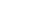since its elements are those of α and α itself.

A nonzero ordinal that is not a successor is called a limit ordinal. One justification for this term is that a limit ordinal is indeed the limit
Limit point
In mathematics, a limit point of a set S in a topological space X is a point x in X that can be "approximated" by points of S in the sense that every neighbourhood of x with respect to the topology on X also contains a point of S other than x itself. Note that x does not have to be an element of S...

in a topological sense of all smaller ordinals (under the order topology
Order topology
In mathematics, an order topology is a certain topology that can be defined on any totally ordered set. It is a natural generalization of the topology of the real numbers to arbitrary totally ordered sets...

).

When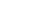is an ordinal-indexed sequence, indexed by a limit γ and the sequence is increasing, i.e.whenever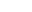we define its limit to be the least upper bound of the set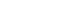that is, the smallest ordinal (it always exists) greater than any term of the sequence. In this sense, a limit ordinal is the limit of all smaller ordinals (indexed by itself). Put more directly, it is the supremum of the set of smaller ordinals.

Another way of defining a limit ordinal is to say that α is a limit ordinal if and only if:
There is an ordinal less than α and whenever ζ is an ordinal less than α, then there exists an ordinal ξ such that ζ < ξ < α.

So in the following sequence:
0, 1, 2, ... , ω, ω+1

ω is a limit ordinal because for any smaller ordinal (in this example, a natural number) we can find another ordinal (natural number) larger than it, but still less than ω.

Thus, every ordinal is either zero, or a successor (of a well-defined predecessor), or a limit. This distinction is important, because many definitions by transfinite induction rely upon it. Very often, when defining a function F by transfinite induction on all ordinals, one defines F(0), and F(α+1) assuming F(α) is defined, and then, for limit ordinals δ one defines F(δ) as the limit of the F(β) for all β<δ (either in the sense of ordinal limits, as we have just explained, or for some other notion of limit if F does not take ordinal values). Thus, the interesting step in the definition is the successor step, not the limit ordinals. Such functions (especially for F nondecreasing and taking ordinal values) are called continuous. We will see that ordinal addition, multiplication and exponentiation are continuous as functions of their second argument.

### Indexing classes of ordinals

We have mentioned that any well-ordered set is similar (order-isomorphic) to a unique ordinal number, or, in other words, that its elements can be indexed in increasing fashion by the ordinals less than. This applies, in particular, to any set of ordinals: any set of ordinals is naturally indexed by the ordinals less than some. The same holds, with a slight modification, for classes of ordinals (a collection of ordinals, possibly too large to form a set, defined by some property): any class of ordinals can be indexed by ordinals (and, when the class is unbounded in the class of all ordinals, this puts it in class-bijection with the class of all ordinals). So we can freely speak of the-th element in the class (with the convention that the “0-th” is the smallest, the “1-th” is the next smallest, and so on). Formally, the definition is by transfinite induction: the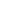-th element of the class is defined (provided it has already been defined for all), as the smallest element greater than the-th element for all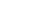.

We can apply this, for example, to the class of limit ordinals: the-th ordinal, which is either a limit or zero is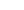(see ordinal arithmetic
Ordinal arithmetic
In the mathematical field of set theory, ordinal arithmetic describes the three usual operations on ordinal numbers: addition, multiplication, and exponentiation. Each can be defined in essentially two different ways: either by constructing an explicit well-ordered set which represents the...

for the definition of multiplication of ordinals). Similarly, we can consider additively indecomposable ordinal
In set theory, a branch of mathematics, an additively indecomposable ordinal α is any ordinal number that is not 0 such that for any \beta,\gamma...

s
(meaning a nonzero ordinal that is not the sum of two strictly smaller ordinals): the-th additively indecomposable ordinal is indexed as. The technique of indexing classes of ordinals is often useful in the context of fixed points: for example, the-th ordinalsuch that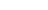is written. These are called the "epsilon number
Epsilon nought
In mathematics, the epsilon numbers are a collection of transfinite numbers whose defining property is that they are fixed points of an exponential map. Consequently, they are not reachable from 0 via a finite series of applications of the chosen exponential map and of "weaker" operations like...

s".

### Closed unbounded sets and classes

A class of ordinals is said to be unbounded, or cofinal, when given any ordinal, there is always some element of the class greater than it (then the class must be a proper class, i.e., it cannot be a set). It is said to be closed when the limit of a sequence of ordinals in the class is again in the class: or, equivalently, when the indexing (class-)function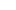is continuous in the sense that, for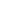a limit ordinal,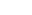(the-th ordinal in the class) is the limit of allfor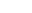; this is also the same as being closed, in the topological
Topological space
Topological spaces are mathematical structures that allow the formal definition of concepts such as convergence, connectedness, and continuity. They appear in virtually every branch of modern mathematics and are a central unifying notion...

sense, for the order topology
Order topology
In mathematics, an order topology is a certain topology that can be defined on any totally ordered set. It is a natural generalization of the topology of the real numbers to arbitrary totally ordered sets...

(to avoid talking of topology on proper classes, one can demand that the intersection of the class with any given ordinal is closed for the order topology on that ordinal, this is again equivalent).

Of particular importance are those classes of ordinals that are closed and unbounded
Club set
In mathematics, particularly in mathematical logic and set theory, a club set is a subset of a limit ordinal which is closed under the order topology, and is unbounded relative to the limit ordinal...

, sometimes called clubs. For example, the class of all limit ordinals is closed and unbounded: this translates the fact that there is always a limit ordinal greater than a given ordinal, and that a limit of limit ordinals is a limit ordinal (a fortunate fact if the terminology is to make any sense at all!). The class of additively indecomposable ordinals, or the class of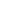ordinals, or the class of cardinals, are all closed unbounded; the set of regular cardinals, however, is unbounded but not closed, and any finite set of ordinals is closed but not unbounded.

A class is stationary if it has a nonempty intersection with every closed unbounded class. All superclasses of closed unbounded classes are stationary, and stationary classes are unbounded, but there are stationary classes that are not closed and stationary classes that have no closed unbounded subclass (such as the class of all limit ordinals with countable cofinality). Since the intersection of two closed unbounded classes is closed and unbounded, the intersection of a stationary class and a closed unbounded class is stationary. But the intersection of two stationary classes may be empty, e.g. the class of ordinals with cofinality ω with the class of ordinals with uncountable cofinality.

Rather than formulating these definitions for (proper) classes of ordinals, we can formulate them for sets of ordinals below a given ordinal: A subset of a limit ordinalis said to be unbounded (or cofinal) underprovided any ordinal less thanis less than some ordinal in the set. More generally, we can call a subset of any ordinalcofinal inprovided every ordinal less thanis less than or equal to some ordinal in the set. The subset is said to be closed underprovided it is closed for the order topology in, i.e. a limit of ordinals in the set is either in the set or equal toitself.

## Arithmetic of ordinals

There are three usual operations on ordinals: addition, multiplication, and (ordinal) exponentiation. Each can be defined in essentially two different ways: either by constructing an explicit well-ordered set that represents the operation or by using transfinite recursion. Cantor normal form provides a standardized way of writing ordinals. The so-called "natural" arithmetical operations retain commutativity at the expense of continuity.

### Initial ordinal of a cardinal

Each ordinal has an associated cardinal
Cardinal number
In mathematics, cardinal numbers, or cardinals for short, are a generalization of the natural numbers used to measure the cardinality of sets. The cardinality of a finite set is a natural number – the number of elements in the set. The transfinite cardinal numbers describe the sizes of infinite...

, its cardinality, obtained by simply forgetting the order. Any well-ordered set having that ordinal as its order-type has the same cardinality. The smallest ordinal having a given cardinal as its cardinality is called the initial ordinal of that cardinal. Every finite ordinal (natural number) is initial, but most infinite ordinals are not initial. The axiom of choice is equivalent to the statement that every set can be well-ordered, i.e. that every cardinal has an initial ordinal. In this case, it is traditional to identify the cardinal number with its initial ordinal, and we say that the initial ordinal is a cardinal.

Cantor used the cardinality to partition ordinals into classes. He referred to the natural numbers as the first number class, the ordinals with cardinality ℵ0 (the countably infinite ordinals) as the second number class and generally, the ordinals with cardinality ℵn−2 as the n-th number class.

The α-th infinite initial ordinal is written. Its cardinality is written ℵα. For example, the cardinality of ω0 = ω is ℵ0, which is also the cardinality of ω2 or ε0 (all are countable ordinals). So (assuming the axiom of choice) we identify ω with ℵ0, except that the notation ℵ0 is used when writing cardinals, and ω when writing ordinals (this is important since, for example, whereas). Also,is the smallest uncountable ordinal (to see that it exists, consider the set of equivalence classes of well-orderings of the natural numbers: each such well-ordering defines a countable ordinal, andis the order type of that set),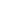is the smallest ordinal whose cardinality is greater than ℵ1, and so on, andis the limit of thefor natural numbers n (any limit of cardinals is a cardinal, so this limit is indeed the first cardinal after all the).

Von Neumann cardinal assignment
The von Neumann cardinal assignment is a cardinal assignment which uses ordinal numbers. For a well-ordered set U, we define its cardinal number to be the smallest ordinal number equinumerous to U. More precisely:...

.

### Cofinality

The cofinality
Cofinality
In mathematics, especially in order theory, the cofinality cf of a partially ordered set A is the least of the cardinalities of the cofinal subsets of A....

of an ordinalis the smallest ordinalthat is the order type of a cofinal
Cofinal (mathematics)
In mathematics, let A be a set and let ≤ be a binary relation on A. Then a subset B of A is said to be cofinal if it satisfies the following condition:This definition is most commonly applied when A is a partially ordered set or directed set under the relation ≤. Also, the notion of cofinal...

subset of. Notice that a number of authors define cofinality or use it only for limit ordinals. The cofinality of a set of ordinals or any other well ordered set is the cofinality of the order type of that set.

Thus for a limit ordinal, there exists a-indexed strictly increasing sequence with limit. For example, the cofinality of ω² is ω, because the sequence ω·m (where m ranges over the natural numbers) tends to ω²; but, more generally, any countable limit ordinal has cofinality ω. An uncountable limit ordinal may have either cofinality ω as does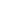or an uncountable cofinality.

The cofinality of 0 is 0. And the cofinality of any successor ordinal is 1. The cofinality of any limit ordinal is at least.

An ordinal that is equal to its cofinality is called regular and it is always an initial ordinal. Any limit of regular ordinals is a limit of initial ordinals and thus is also initial even if it is not regular, which it usually is not. If the Axiom of Choice, then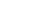is regular for each α. In this case, the ordinals 0, 1,,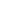, andare regular, whereas 2, 3,, and ωω·2 are initial ordinals that are not regular.

The cofinality of any ordinal α is a regular ordinal, i.e. the cofinality of the cofinality of α is the same as the cofinality of α. So the cofinality operation is idempotent.

## Some “large” countable ordinals

We have already mentioned (see Cantor normal form) the ordinal ε0, which is the smallest satisfying the equation, so it is the limit of the sequence 0, 1,,,, etc. Many ordinals can be defined in such a manner as fixed points of certain ordinal functions (the-th ordinal such thatis called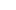, then we could go on trying to find the-th ordinal such that, “and so on”, but all the subtlety lies in the “and so on”). We can try to do this systematically, but no matter what system is used to define and construct ordinals, there is always an ordinal that lies just above all the ordinals constructed by the system. Perhaps the most important ordinal that limits a system of construction in this manner is the Church–Kleene ordinal,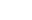(despite thein the name, this ordinal is countable), which is the smallest ordinal that cannot in any way be represented by a computable function
Computable function
Computable functions are the basic objects of study in computability theory. Computable functions are the formalized analogue of the intuitive notion of algorithm. They are used to discuss computability without referring to any concrete model of computation such as Turing machines or register...

(this can be made rigorous, of course). Considerably large ordinals can be defined below, however, which measure the “proof-theoretic strength” of certain formal system
Formal system
In formal logic, a formal system consists of a formal language and a set of inference rules, used to derive an expression from one or more other premises that are antecedently supposed or derived . The axioms and rules may be called a deductive apparatus...

s (for example,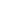measures the strength of Peano arithmetic
Peano axioms
In mathematical logic, the Peano axioms, also known as the Dedekind–Peano axioms or the Peano postulates, are a set of axioms for the natural numbers presented by the 19th century Italian mathematician Giuseppe Peano...

). Large ordinals can also be defined above the Church-Kleene ordinal, which are of interest in various parts of logic.

## Topology and ordinals

Any ordinal can be made into a topological space
Topological space
Topological spaces are mathematical structures that allow the formal definition of concepts such as convergence, connectedness, and continuity. They appear in virtually every branch of modern mathematics and are a central unifying notion...

in a natural way by endowing it with the order topology
Order topology
In mathematics, an order topology is a certain topology that can be defined on any totally ordered set. It is a natural generalization of the topology of the real numbers to arbitrary totally ordered sets...

.
See the Topology and ordinals section of the "Order topology" article.

## Downward closed sets of ordinals

A set is downward closed if anything less than an element of the set is also in the set. If a set of ordinals is downward closed, then that set is an ordinal—the least ordinal not in the set.

Examples:
• The set of ordinals less than 3 is 3 = { 0, 1, 2 }, the smallest ordinal not less than 3.
• The set of finite ordinals is infinite, the smallest infinite ordinal: ω.
• The set of countable ordinals is uncountable, the smallest uncountable ordinal: ω1.